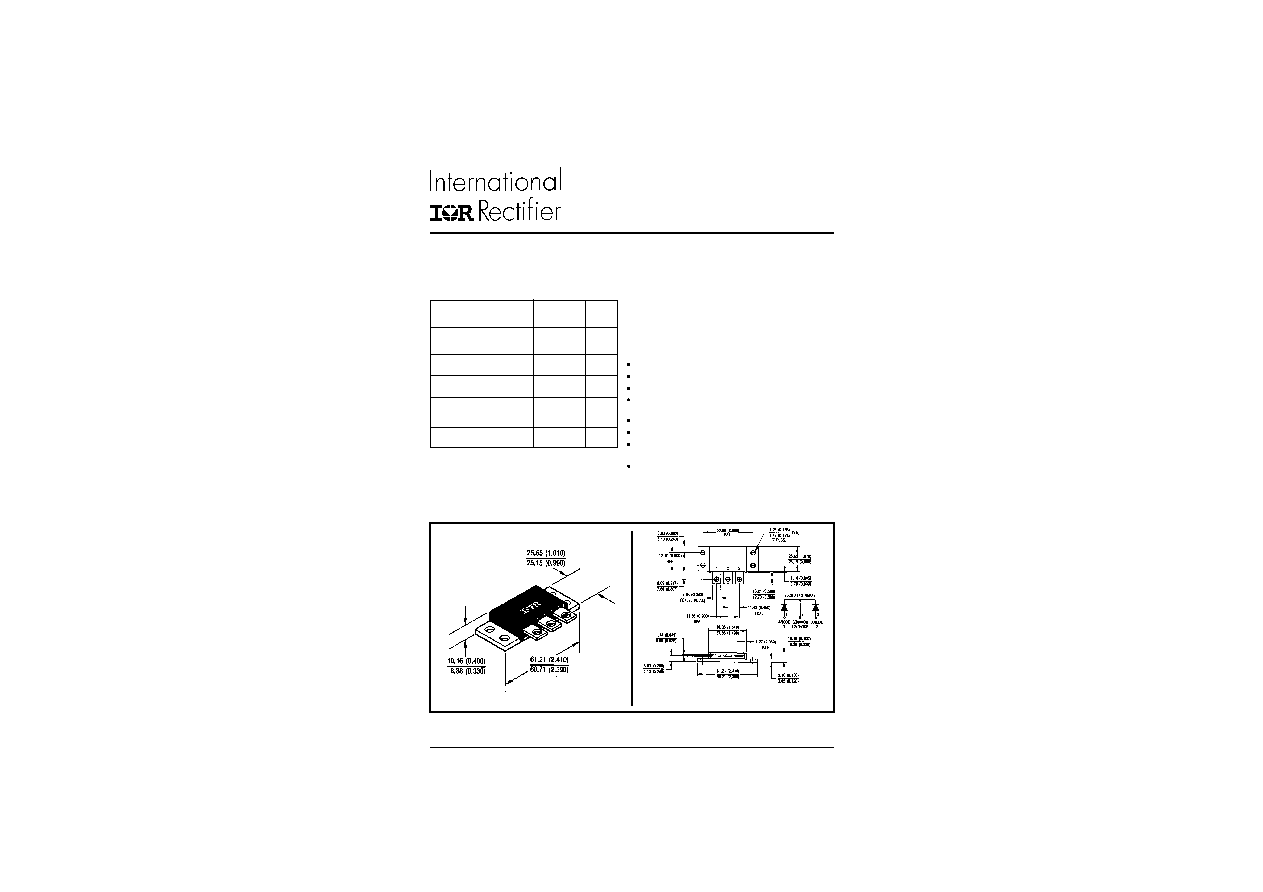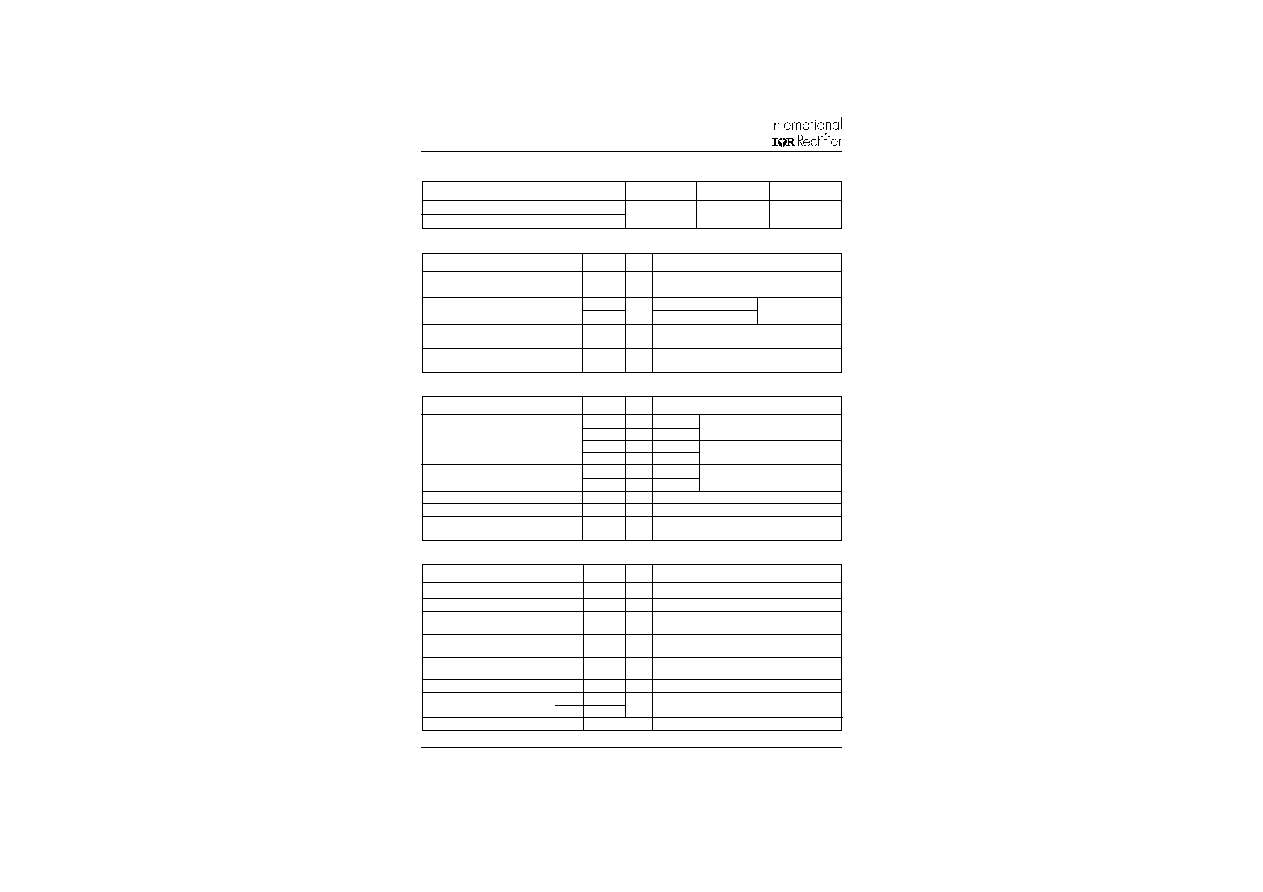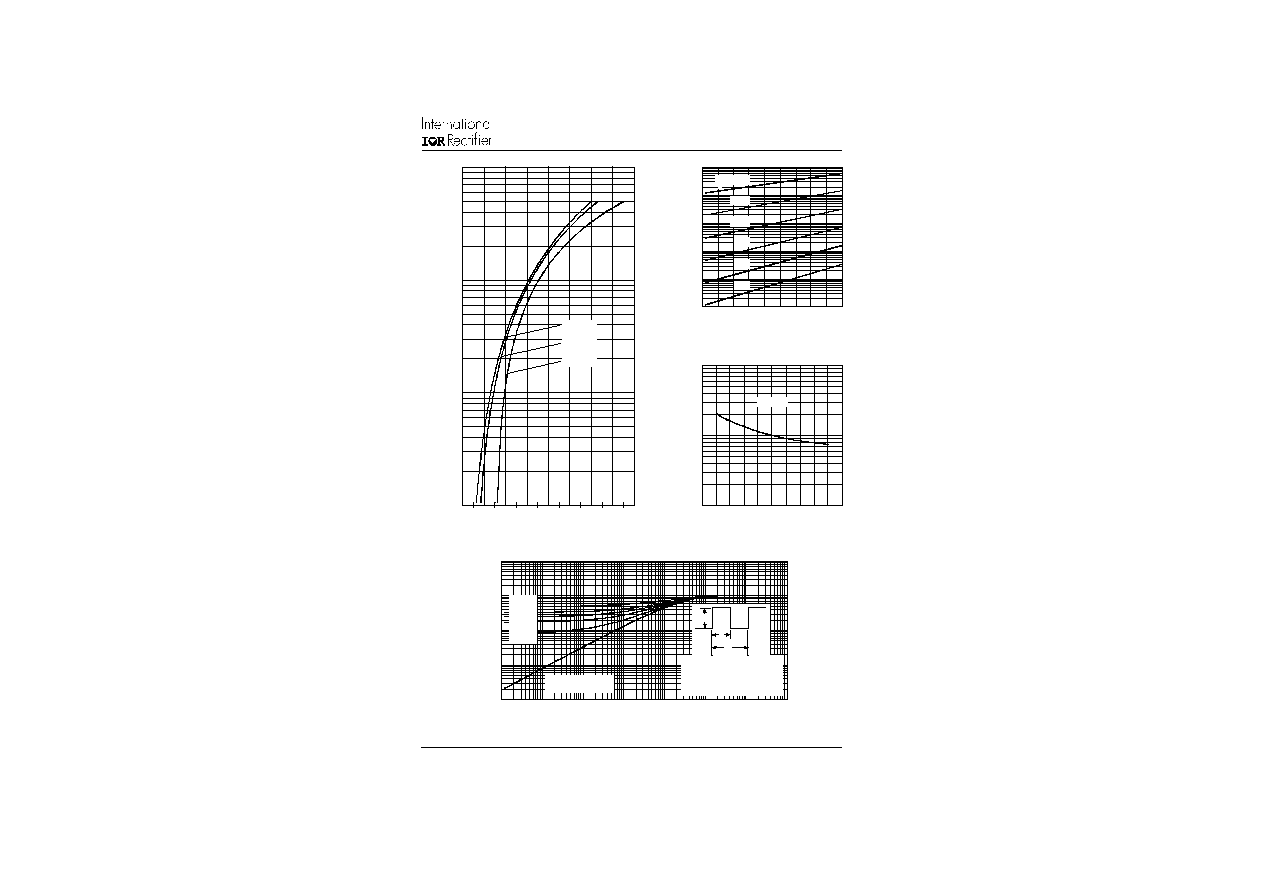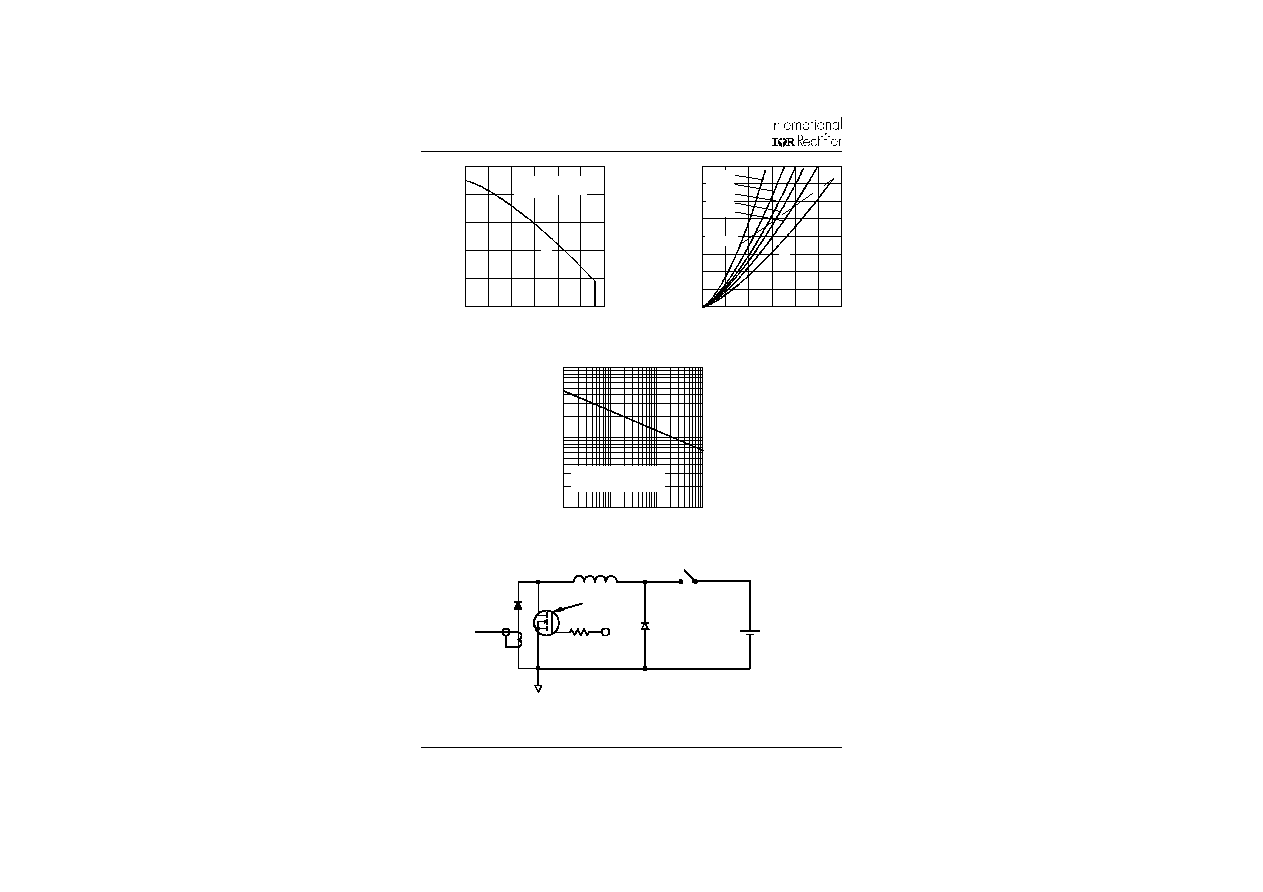Search datasheet (1.687.043 components) Search fieldPart namePart descriptionAbout site Manufacturers list ChipFindIC search engine AllXrefCross-reference database### Datasheet: 160CMQ045 (International Rectifier)

SCHOTTKY RECTIFIER
Download:PDFZIPSCHOTTKY RECTIFIER
160 Amp
160CMQ... SERIES
www.irf.com
1
PD-2.254 rev. A 12/97
CASE STYLE AND DIMENSIONS
Conforms to JEDEC Outline TO - 249AA
Dimensions in millimeters and inches
Major Ratings and Characteristics
I
F(AV)
Rectangular
160
A
waveform
V
RRM
range
35 to 45
V
I
FSM
@ tp = 5 �s sine
6400
A
V
F
@
80 Apk, T
J
= 125�C
0.60
V
(per leg)
T
J
range
- 55 to 150
�C
Characteristics
160CMQ... Units
The 160CMQ isolated, center tap Schottky rectifier module
series has been optimized for very low forward voltage drop,
with moderate leakage. The proprietary barrier technology
allows for reliable operation up to 150� C junction temperature.
Typical applications are in switching power supplies, convert-
ers, free-wheeling diodes, and reverse battery protection.
150� C T
J
operation
Isolated heatsink
Center tap module
High purity, high temperature epoxy encapsulation for
enhanced mechanical strength and moisture resistance
Very low forward voltage drop
High frequency operation
Guard ring for enhanced ruggedness and long term
reliability
Low profile, high current package
Description/Features160CMQ... Series
2
PD-2.254 rev. A 12/97
www.irf.com
T
J
Max. Junction Temperature Range
-55 to 150
�C
T
stg
Max. Storage Temperature Range
-55 to 150
�C
R
thJC
Max. Thermal Resistance Junction
1.0
�C/W DC operation
* See Fig. 4
to Case (Per Leg)
R
thJC
Max. Thermal Resistance Junction
0.50
�C/W DC operation
to Case (Per Package)
R
thCS
Typical Thermal Resistance, Case
0.10
�C/W Mounting surface , smooth and greased
to Heatsink
wt
Approximate Weight
58 (2.0)
g (oz.)
T
Mounting Torque
Min.
40 (35)
Max.
58 (50)
Case Style
TO - 249AA
JEDEC
Thermal-Mechanical Specifications
Kg-cm
(Ibf-in)
V
FM
Max. Forward Voltage Drop
0.64
V
@ 80A
(Per Leg) * See Fig. 1
(1)
0.86
V
@ 160A
0.60
V
@ 80A
0.76
V
@ 160A
I
RM
Max. Reverse Leakage Current
5
mA
T
J
= 25 �C
(Per Leg) * See Fig. 2
(1)
200
mA
T
J
= 125 �C
C
T
Max. Junction Capacitance (Per Leg)
2600
pF
V
R
= 5V
DC
, (test signal range 100Khz to 1Mhz) 25�C
L
S
Typical Series Inductance (Per Leg)
8.0
nH
Measured from terminal hole to terminal hole
dv/dt Max. Voltage Rate of Change
10,000
V/ �s
(Rated V
R
)
T
J
= 25 �C
T
J
= 125 �C
Electrical Specifications
(1) Pulse Width < 300�s, Duty Cycle <2%
V
R
= rated V
R
Absolute Maximum Ratings
Following any rated
load condition and with
rated V
RRM
applied
Parameters
160CMQ Units
Conditions
I
F(AV)
Max. Average Forward Current
160
A
50% duty cycle @ T
C
= 69 �C, rectangular wave form
* See Fig. 5
I
FSM
Max. Peak One Cycle Non-Repetitive
6400
5�s Sine or 3�s Rect. pulse
Surge Current (Per Leg) * See Fig. 7
750
10ms Sine or 6ms Rect. pulse
E
AS
Non-Repetitive Avalanche Energy
108
mJ
T
J
= 25 �C, I
AS
= 16 Amps, L = 0.84 mH
(Per Leg)
I
AR
Repetitive Avalanche Current
16
A
Current decaying linearly to zero in 1 �sec
(Per Leg)
Frequency limited by T
J
max. V
A
= 1.5 x V
R
typical
A
Part number
160CMQ035
160CMQ040
160CMQ045
V
R
Max. DC Reverse Voltage (V)
V
RWM
Max. Working Peak Reverse Voltage (V)
Voltage Ratings
35
40
45
Parameters
160CMQ Units
Conditions
Parameters
160CMQ Units
Conditions160CNQ... Series
3
PD-2.254 rev. A
12/97
www.irf.com
Fig. 2 - Typical Values Of Reverse Current
Vs. Reverse Voltage (Per Leg)
Fig. 3 - Typical Junction Capacitance
Vs. Reverse Voltage (Per Leg)
Fig. 4 - Max. Thermal Impedance Z
thJC
Characteristics (Per Leg)
.0
1
.1
1
10
10
0
10
00
0
5
10
15
2
0
25
30
3
5
4
0
4
5
R
R
12
5
�C
10
0�
C
75

C
50

C
25

C
R
ev
er
s
e
V
o
l
t
ag
e -
V

(
V
)
Reverse Current - I (mA)
T

=

15
0�
C
J
.
001
.0
1
.1
1
10
.
0
000
1
.
0
0
0
1
.
0
0
1
.
0
1
.
1
1
10
10
0
D
=
0
.
3
3
D
=
0
.
5
0
D
=
0
.
2
5
D
=
0
.
1
7
D
=
0
.
0
8
1
thJC
t
,

R
e
c
t
an
gul
ar
P
u
l
s
e D
ur
at
i
on (
S
e
c
on
ds
)
Thermal Impedance - Z (�C/W
)
S
i
n
g
l
e
P
u
l
s
e

(T
h
e
rm
a
l
R
e
si
st
a
n
ce
)
2 t
1 t
P
D
M
No
t
e
s
:
1.
D
u
t
y

fa
c
t
o
r

D
=

t
/ t
2
.
P
e
a
k

T

=

P

x
Z
+
T
1
JD
M
th
J
C
C
2
Fig. 1 - Max. Forward Voltage Drop Characteristics
(Per Leg)
1
10
10
0
10
00
0
.
2.
4
.
6.
8
1
1
.
2
1
.
4
1
.
6
FM
F
Instantaneous Forward Current - I (A)
F
o
r
w
a
r
d
V
o
l
t
ag
e D
r
op
-
V

(
V
)
T
=
1
5
0
�C
T

=
12
5�
C
T
=
2
5

C
J
J
J
10
0
10
00
1
000
0
0
1
02
03
04
05
0
T
=
2
5
�C
J
R
ev
e
r
s
e
V
o
l
t
ag
e
-

V
(
V
)
R
T
Junction Capacitance - C (pF)160CMQ... Series
4
PD-2.254 rev. A
12/97
www.irf.com
Fig. 7 - Max. Non-Repetitive Surge Current (Per Leg)
Fig. 5 - Max. Allowable Case Temperature
Vs. Average Forward Current (Per Leg)
Fig. 8 - Unclamped Inductive Test Circuit
Fig. 6 - Forward Power Loss Characteristics
(Per Leg)
0
10
20
30
40
50
60
70
80
0
2
0
4
06
08
0
1
0
0
1
2
0
DC
Average Power Loss - (W
atts)
F
(
AV)
D
=
0.
08
D
=
0.
17
D
=
0.
25
D
=
0.
33
D
=
0.
50
R
M
S L
i
m
it
A
v
er
ag
e
F
o
r
w
ar
d
C
u
r
r
e
n
t
-

I
(
A
)
60
80
10
0
12
0
14
0
16
0
0
2
04
06
08
0
1
0
0
1
2
0
DC
Allowable Case Temperature - (�C)
A
v
er
ag
e F
o
r
w
ar
d C
u
r
r
e
n
t
-
I
(
A
)
F
(
AV)
16
0C
MQ
R

(
D
C
) =

1
.
0
�C
/
W
th
J
C
10
0
10
00
1
000
0
1
0
100
1
000
10
000
FSM
p
Non-Repetitive Surge Current - I (A)
A
t
A
n
y
R
a
t
ed L
C
o
n
d
i
t
i
o
n
A
n
d

W
i
th
R
a
t
e
d

V

A
p
p
l
i
e
d
F
o
l
l
o
w
i
ng S
u
r
g
e
RR
M
S
quar
e
W
a
v
e
P
u
l
s
e
D
u
r
a
t
i
on -
t
(
m
i
c
r
o
s
e
c
)
F
R
EE-
W
H
EEL

D
I
O
D
E
40
H
F
L
4
0
S
02
C
U
RRE
N
T
MO
N
I
T
O
R
HI
G
H
-
S
P
E
ED

S
W
I
T
C
H
IR
F
P
4
6
0
L
DU
T
R
g
=

25
o
h
m
Vd
=
2
5
Vo
l
t
+© 2019 • ICSheet• Contact form• Main page